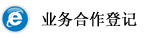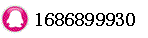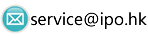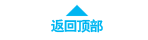# Shareholder Equity Ratio时间：2018-05-02 21:21:58     浏览：203    评论：0

A ratio used to help determine how much shareholders would receive in the event of a company-wide liquidation. The ratio, expressed as a percentage, is calculated by dividing total shareholders' equity by total assets of the firm, and it represents the amount of assets on which shareholders have a residual claim. The figures used to calculate the ratio are taken from the company's balance sheet.

Taobiz explains Shareholder Equity Ratio
For example, let's say a company has a shareholder equity ratio of 45% and total assets of \$500 million. This would mean that, in the event of liquidation, all shareholders would receive \$225 million . The higher the ratio, the more shareholders may receive - and of course, the reverse holds true.

0相关评论联系我们